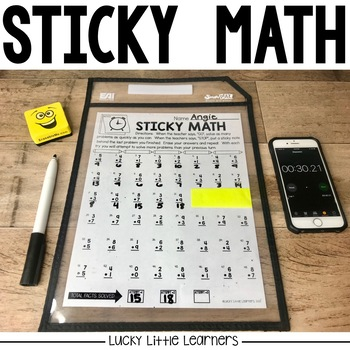# Sticky Math Bundle | Math Fact Fluency PracticeGrade Levels
1st - 2nd
Subjects
Standards
Resource Type
Formats Included
• Zip
Pages
189 pages
\$8.00
Bundle
List Price:
\$10.00
You Save:
\$2.00
\$8.00
Bundle
List Price:
\$10.00
You Save:
\$2.00
Share this resource

### Description

This BUNDLED resource includes both the original Sticky Math program with the additional Sticky Math sheets for a total of 4 Sticky Math sheets for each level.

Say goodbye to timed tests that stress students and teachers out! No more correcting, no more copies, no more stress.

Sticky Math is a fun and new way for your students to practice their math facts in a developmentally appropriate and self motivating way!

Students receive a sticky math sheet. The students are racing against themselves and don't have to share their scores with the rest of the class.

When the teacher says "go" the students solve the problems as quickly as they can. When the teachers says "stop" the students place their sticky note on the last problem that they completed. This process is repeated two more times. Each time this is done, the students try to beat their previous score.

MATERIALS INCLUDED:

-Student Tracker

-Directions

-Addition Sticky Math Sheets (mental math strategies labeled)

+0, +1, +2, +3, +4, +5, +6, +7, +8, +9, doubles, doubles +1, mixed
(4 options of sticky math sheets for each level!)

-Subtraction Sticky Math Sheets (mental math strategies labeled)

-0, -1, -2, -3, -4, -5, -6, -7, -8, -9, mixed

-Sticky Math Sheet with addition and subtraction facts mixed

(4 options of sticky math sheets for each level!)

-Answer Keys (optional)

MATERIALS NEEDED:

-sheet protectors

-timer

-dry erase marker

-eraser

-sticky notes

▼▼▼▼▼▼▼▼▼▼▼▼▼▼▼▼▼▼▼▼▼▼▼▼▼▼▼▼▼▼▼▼▼▼▼▼▼▼▼▼▼▼▼▼

If you would like to get updates on NEW and CURRENT resources...

▼▼▼▼▼▼▼▼▼▼▼▼▼▼▼▼▼▼▼▼▼▼▼▼▼▼▼▼▼▼▼▼▼▼▼▼▼▼▼▼▼▼▼▼

Copyright © Lucky Little Learners, LLC.

All rights reserved by author.

Permission to copy for single classroom use only.

Electronic distribution limited to single classroom use only.

Not for public display.

If you have any questions about this resource, please contact me at customerservice@luckylittlelearners.com

Total Pages
189 pages
Answer Key
Included
Teaching Duration
Other
Report this Resource to TpT
Reported resources will be reviewed by our team. Report this resource to let us know if this resource violates TpT’s content guidelines.

### Standards

to see state-specific standards (only available in the US).
Fluently add and subtract within 20 using mental strategies. By end of Grade 2, know from memory all sums of two one-digit numbers.
Add and subtract within 20, demonstrating fluency for addition and subtraction within 10. Use strategies such as counting on; making ten (e.g., 8 + 6 = 8 + 2 + 4 = 10 + 4 = 14); decomposing a number leading to a ten (e.g., 13 - 4 = 13 - 3 - 1 = 10 - 1 = 9); using the relationship between addition and subtraction (e.g., knowing that 8 + 4 = 12, one knows 12 - 8 = 4); and creating equivalent but easier or known sums (e.g., adding 6 + 7 by creating the known equivalent 6 + 6 + 1 = 12 + 1 = 13).
Relate counting to addition and subtraction (e.g., by counting on 2 to add 2).
Understand subtraction as an unknown-addend problem. For example, subtract 10 – 8 by finding the number that makes 10 when added to 8.
Apply properties of operations as strategies to add and subtract. If 8 + 3 = 11 is known, then 3 + 8 = 11 is also known. (Commutative property of addition.) To add 2 + 6 + 4, the second two numbers can be added to make a ten, so 2 + 6 + 4 = 2 + 10 = 12. (Associative property of addition.)

### Questions & Answers

Teachers Pay Teachers is an online marketplace where teachers buy and sell original educational materials.

More About Us